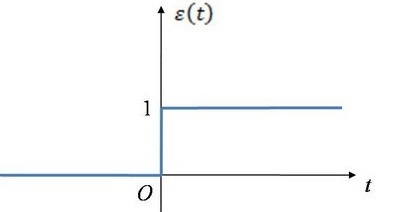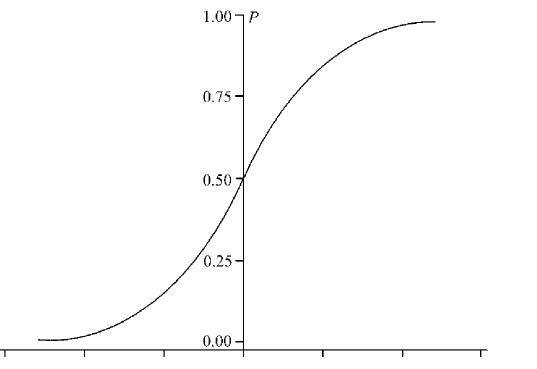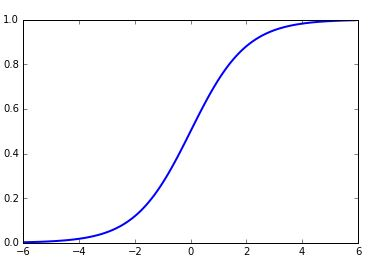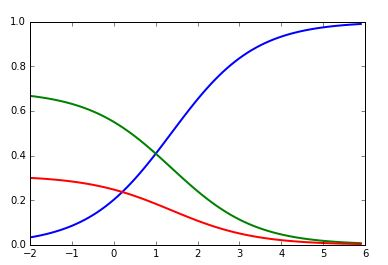# python:logistic,softmax函数logistic函数：一种常见的S形函数，它是皮埃尔·弗朗索瓦·韦吕勒在1844或1845年在研究它与人口增长的关系时命名的。（生物课上经常看到）g(z)=11+ez

logistic函数的优点：

python实现：

import numpy as np
import matplotlib.pyplot as plt
def logistic(x):
return 1/(1+np.exp(-x))

x=np.arange(-6.0,6.0,0.1)
plt.plot(x,logistic(x).T,linewidth=2)
plt.show()softmax函数：

softmax函数公式：

σ(z)j=ezjKk=1ezk

python代码：

import numpy as np
import matplotlib.pyplot as plt

def softmax(x):
return np.exp(x)/np.sum(np.exp(x),axis=0)

x=np.arange(-2.0,6.0,0.1)
scores=np.stack([x,np.ones_like(x),0.2*np.ones_like(x)])
plt.plot(x,softmax(scores).T,linewidth=2)
plt.show()logistic函数与softmax函数的区别：
softmax建模使用的分布是多项式分布，而logistic则基于伯努利分布，

pn={1ppn=0n=1

softmax回归和多个logistic回归的关系：
softmax回归进行的多分类，类与类之间是互斥的，即一个输入只能被归为一类；

logistic函数和softmax函数08-2612-121526
09-172146
11-034730
03-031万+
08-136599
11-061664
05-20874
12-102万+
08-10991
09-0787
04-301278
04-081818
12-043326
12-111170
04-241734
12-122万+
03-024657
©️2020 CSDN 皮肤主题: 编程工作室 设计师:CSDN官方博客